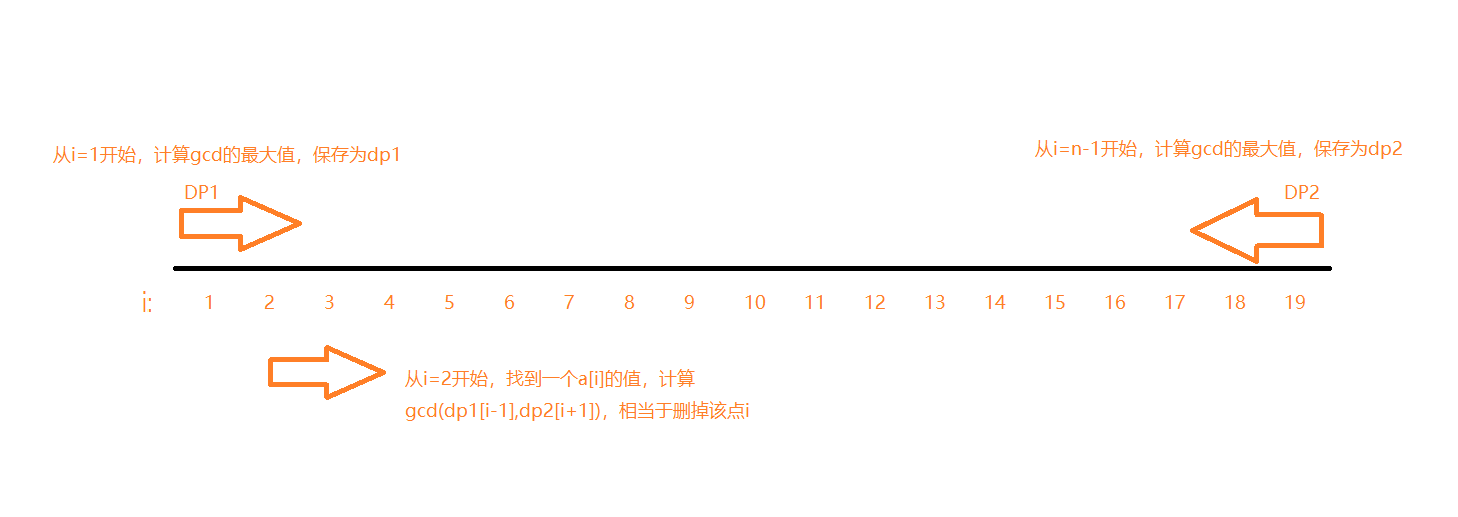## ccpc 女生专场 C - Coprime Sequence HDU - 6025

Do you know what is called Coprime Sequence''? That is a sequence consists of  nnpositive integers, and the GCD (Greatest Common Divisor) of them is equal to 1.
Coprime Sequence'' is easy to find because of its restriction. But we can try to maximize the GCD of these integers by removing exactly one integer. Now given a sequence, please maximize the GCD of its elements.

InputThe first line of the input contains an integer T(1T10)T(1≤T≤10), denoting the number of test cases.
In each test case, there is an integer n(3n100000)n(3≤n≤100000) in the first line, denoting the number of integers in the sequence.
Then the following line consists of nn integers a1,a2,...,an(1ai109)a1,a2,...,an(1≤ai≤109), denoting the elements in the sequence.
OutputFor each test case, print a single line containing a single integer, denoting the maximum GCD.Sample Input

3
3
1 1 1
5
2 2 2 3 2
4
1 2 4 8

Sample Output

1
2
2#include<iostream>
#include<cstdio>
#include<cstring>
#include<string>
#include<algorithm>
#include<cmath>
#include<utility>
#include<set>
#include<vector>
#include<map>
#include<queue>
#include<stack>
#define maxn 110
#define INF 0x3f3f3f3f
#define LL long long
#define ULL unsigned long long
#define E 1e-8
#define mod 100000000
using namespace std;
#define raf(i,k,n) for(int i=k;i<=n;i++)
//Oo0Oooo00ooOoo00oO
int gcd(int a,int b)
{
return b==0?a:gcd(b,a%b);
}
int a;
int dp1,dp2;
int main()
{
int t;
cin>>t;
while(t--)
{
int n;
cin>>n;
for(int i = 0 ; i < n ; i++ )   cin>>a[i];
dp1  = a;
for(int i = 1 ; i < n ; i++ )   dp1[i] = gcd(dp1[i-1],a[i]);
dp2[n-1] = a[n-1];
for(int i = n-2 ; i >= 0 ; i--)  dp2[i] = gcd(dp2[i+1],a[i]);
int ma = max(dp2,dp1[n-2]);
//cout<<"dp2 "<<dp2[n-2];
for(int i=1;i<n-1;i++)

ma = max(gcd(dp1[i-1] , dp2[i+1] ), ma);

cout<<ma<<endl;
}
return 0;
}